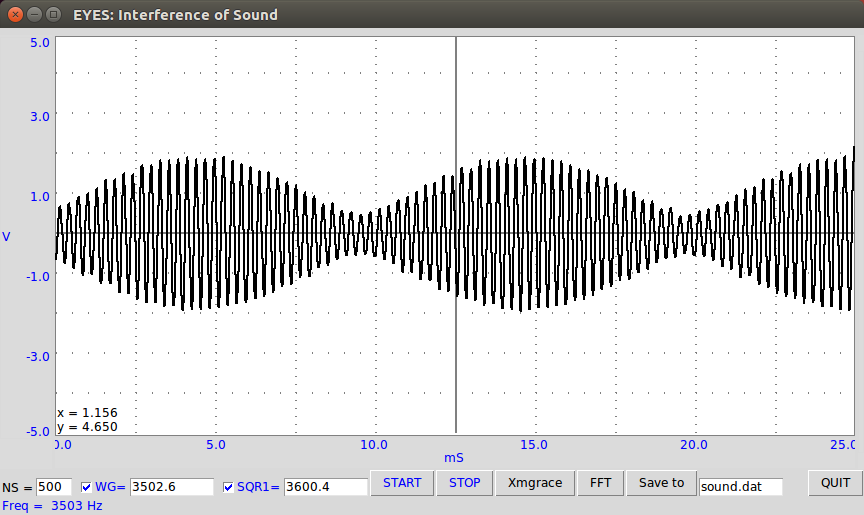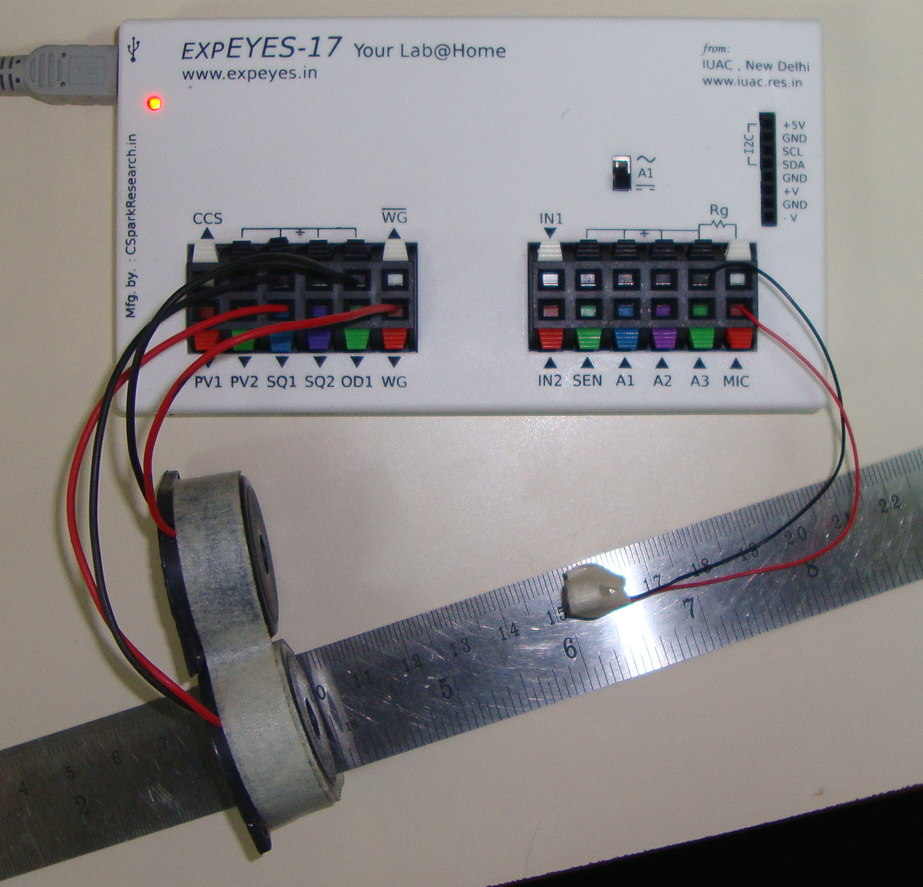# Interference of Sound

## Observation and analysis of Beats phenomena through ExperimentsBrief Summary
When two sound waves with frequencies near each other overlap, constructive and destructive interference causes a third frequency to be observed with a frequency equal to the difference between the two.

## Introduction

Two Piezo buzzers are connected to SQ1, and WG . One is driven with a frequency of 3000Hz, and the other with a nearby frequency such as 2900 Hz. The combination results in a ringing sound which has a frequency equal to 3000 - 2900 . This is called the ‘beats’ phenomenon, and is a result of interference between the two waves.

The oscilloscope monitors three signals:

• the waves driving the Piezos. WG is connected to A1, and SQ1 to A2. WG and SQ1 are also connected to one piezo each
• and the signal detected by the microphone.The microphone is used to digitize this new sound, in order to see what the signal looks like

### ScreenshotYou can observe that the higher frequency now has an oscillating envelope. The frequency of this envelope can be calculated by measuring the time between each node, and taking the reciprocal.

e.g : If two nodes are formed at 10mS, and 20mS positions, the time period of the beat is 10mS. This corresponds to a frequency of 100Hz !

The resonance frequecy of the Piezo disc supplied with ExpEYES is around 3500 Hz. It could vary from unit to unit, so as you move away from that frequency, the loudness will also decrease.

## Fourier transform

A fourier transform of this signal reveals the frequencies of the initial components which were used to create it.

## Exercises:

• Use different values for SQ1 , and WG , and measure the time period. Is it equal to the time period of SQ1-WG?